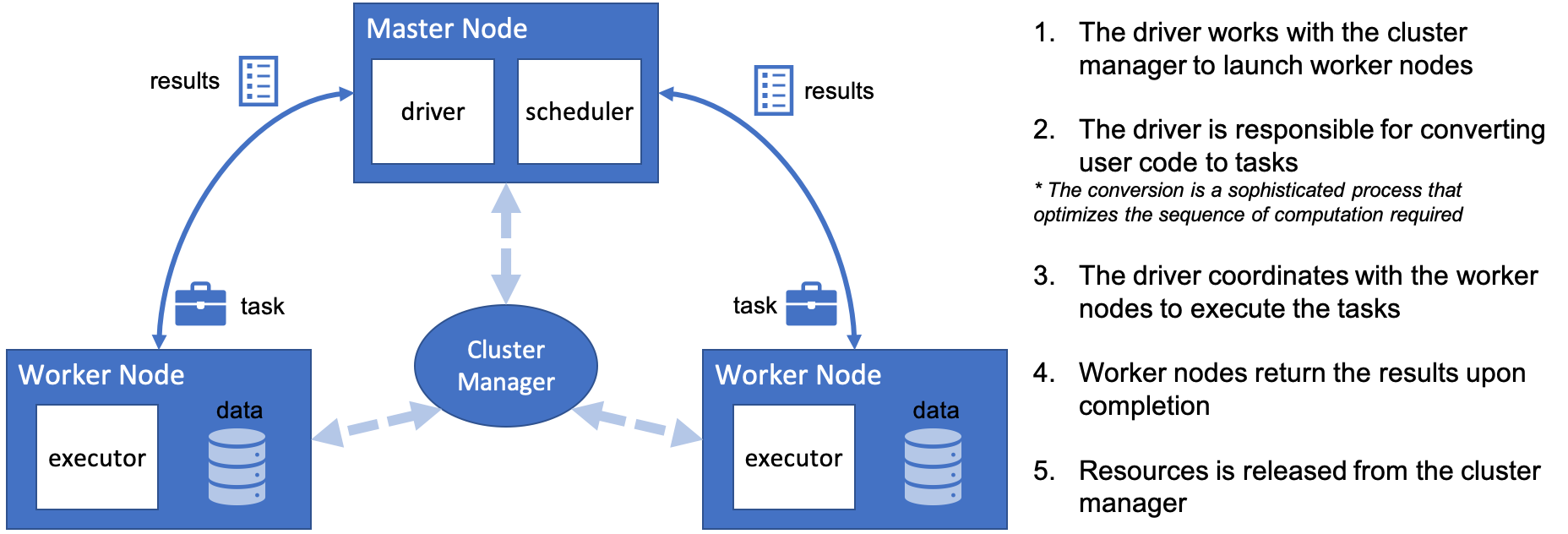1594662300

A Quick Way to Reformat Columns in a Pandas DataFrame

A Quick Way to Reformat Columns in a Pandas DataFrame

#pandas #quick #reformat #columns #dataframe

Buddha Community1624431580

How to add a new column to Pandas DataFrame?

In this tutorial, we are going to discuss different ways to add a new column to pandas data frame.

What is a pandas data frame?

Pandas data frameis a two-dimensional heterogeneous data structure that stores the data in a tabular form with labeled indexes i.e. rows and columns.

Usually, data frames are used when we have to deal with a large dataset, then we can simply see the summary of that large dataset by loading it into a pandas data frame and see the summary of the data frame.

In the real-world scenario, a pandas data frame is created by loading the datasets from an existing CSV file, Excel file, etc.

But pandas data frame can be also created from the listdictionary, list of lists, list of dictionaries, dictionary of ndarray/lists, etc. Before we start discussing how to add a new column to an existing data frame we require a pandas data frame.

#pandas #dataframe #pandas dataframe #column #add a new column #how to add a new column to pandas dataframe1594662300

A Quick Way to Reformat Columns in a Pandas DataFrame

A Quick Way to Reformat Columns in a Pandas DataFrame

#pandas #quick #reformat #columns #dataframe1623927960

Pandas DataFrame vs. Spark DataFrame: When Parallel Computing Matters

With Performance Comparison Analysis and Guided Example of Animated 3D Wireframe Plot

Python is famous for its vast selection of libraries and resources from the open-source community. As a Data Analyst/Engineer/Scientist, one might be familiar with popular packages such as NumpyPandasScikit-learnKeras, and TensorFlow. Together these modules help us extract value out of data and propels the field of analytics. As data continue to become larger and more complex, one other element to consider is a framework dedicated to processing Big Data, such as Apache Spark. In this article, I will demonstrate the capabilities of distributed/cluster computing and present a comparison between the Pandas DataFrame and Spark DataFrame. My hope is to provide more conviction on choosing the right implementation.

Pandas DataFrame

Pandas has become very popular for its ease of use. It utilizes DataFrames to present data in tabular format like a spreadsheet with rows and columns. Importantly, it has very intuitive methods to perform common analytical tasks and a relatively flat learning curve. It loads all of the data into memory on a single machine (one node) for rapid execution. While the Pandas DataFrame has proven to be tremendously powerful in manipulating data, it does have its limits. With data growing at an exponentially rate, complex data processing becomes expensive to handle and causes performance degradation. These operations require parallelization and distributed computing, which the Pandas DataFrame does not support.

Introducing Cluster/Distribution Computing and Spark DataFrame

Apache Spark is an open-source cluster computing framework. With cluster computing, data processing is distributed and performed in parallel by multiple nodes. This is recognized as the MapReduce framework because the division of labor can usually be characterized by sets of the mapshuffle, and reduce operations found in functional programming. Spark’s implementation of cluster computing is unique because processes 1) are executed in-memory and 2) build up a query plan which does not execute until necessary (known as lazy execution). Although Spark’s cluster computing framework has a broad range of utility, we only look at the Spark DataFrame for the purpose of this article. Similar to those found in Pandas, the Spark DataFrame has intuitive APIs, making it easy to implement.#pandas dataframe vs. spark dataframe: when parallel computing matters #pandas #pandas dataframe #pandas dataframe vs. spark dataframe #spark #when parallel computing matters1623922440

8 Ways to Filter Pandas Dataframes

A practical guide for efficient data analysis

Pandas is a popular data analysis and manipulation library for Python. The core data structure of Pandas is dataframe which stores data in tabular form with labelled rows and columns.

A common operation in data analysis is to filter values based on a condition or multiple conditions. Pandas provides a variety of ways to filter data points (i.e. rows). In this article, we will cover 8 different ways to filter a dataframe.

We start by importing the libraries.

import numpy as np
import pandas as pd

Let’s create a sample dataframe for the examples.

df = pd.DataFrame({

name':['Jane','John','Ashley','Mike','Emily','Jack','Catlin'],
'ctg':['A','A','C','B','B','C','B'],
'val':np.random.random(7).round(2),
'val2':np.random.randint(1,10, size=7)
})

#python #programming #data-science #ways to filter pandas dataframes #filter pandas dataframes #pandas dataframes1623370500

Practice Problems: How To Join DataFrames in Pandas

Hey - Nick here! This page is a free excerpt from my \$199 course Python for Finance, which is 50% off for the next 50 students.

It’s now time for some practice problems! See below for details on how to proceed.

Course Repository & Practice Problems

All of the code for this course’s practice problems can be found in this GitHub repository.

There are two options that you can use to complete the practice problems:

• Open them in your browser with a platform called Binder using this link (recommended)
• Download the repository to your local computer and open them in a Jupyter Notebook using Anaconda (a bit more tedious)

Note that binder can take up to a minute to load the repository, so please be patient.

Within that repository, there is a folder called starter-files and a folder called finished-files. You should open the appropriate practice problems within the starter-files folder and only consult the corresponding file in the finished-files folder if you get stuck.

The repository is public, which means that you can suggest changes using a pull request later in this course if you’d like.

#dataframes #pandas #practice problems: how to join dataframes in pandas #how to join dataframes in pandas #practice #/pandas/issues.